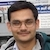# NAEST 2016 Screening Test: Drop Falling in the OilBy

Oil of total mass M is filled into a transparent container. A water drop of mass m is released in the oil container from the top. The drop falls down with its terminal speed. This question from NAEST 2018 asks reaction applied by the bottom of the container on the oil. Also, what is the change in the linear momentum of the oil?

Question 1: Given mass of oil = M and mass of water drop = m. The force by the bottom of the vessel on the oil = N. Assume that the drop moves with the terminal velocity. Choose the correct option(s)

1. $N < (M+m)g$
2. $N = (M+m)g$
3. $N > (M+m)g$
4. The linear momentum of oil is nonzero and is in upward direction.

Solution: Let us analyse this problem in detail. Let density of the oil be $\sigma$ and that of the water be $\rho$. It is easy to see that $\rho>\sigma$ (because drop is falling down in the oil). Let $h$ be the height of oil column (with drop inside it) and $v$ be the terminal velocity of the drop. The volume of water drop is $V=m/\rho$.

Let the origin of the coordinate system be at the point drop's release and $y$-axis be vertically down.Let us consider the oil and the drop together as a system. This system is equivalent to a hypothetical system consisting of the following three components:

1. Oil column of height $h$ (without drop). The mass of this oil column is \begin{align} M_o=M+m(\sigma/\rho) \end{align} The second term is the mass of the oil having the same volume as that of the drop. The centre of mass of this column is $y_\text{cm,o}=h/2$.
2. Water drop of mass $m_d=m$ at a position P with $y$-coordinate $y_\text{cm,d}=y$.
3. A hypothetical drop (cavity) of volume $V=m/\rho$ and density $-\sigma$. The mass of this hypothetical drop is $m_c=-m\sigma/\rho$ and it is co-located with the water drop i.e., $y_\text{cm,c}=y$. Note that the hypothetical drop has a negative mass.

The centre of mass of the system is given by \begin{align} y_{cm}&=\frac{M_o y_\text{cm,o}+m_d y_\text{cm,d}+ m_c y_\text{cm,c}}{M_o+m_d+m_c} \\ &=\frac{(M+m\sigma/\rho)h/2 + m(1-\sigma/\rho)y}{M+m} \end{align} Note that centre of mass of the system moves down as the drop falls ($y$ changes with time). As the drop falls from $y=0$ to $y=h$, the centre of mass of the system moves down a distance $hm(1-\sigma/\rho)/(M+m)$.

The velocity of the centre of mass is given by \begin{align} v_{cm}=\frac{\mathrm{d}y_{cm}}{\mathrm{d}t}=\frac{m(1-\sigma/\rho)v}{M+m} \end{align} The linear momentum of the system is given by \begin{align} p_{cm}=(M+m)v_{cm}=m(1-\sigma/\rho)v \end{align} The linear momentum of the system is constant. Thus, the external force acting on the system must be zero. Hence, the reaction from the bottom of the container must balance the weight i.e., \begin{align} N=(M+m)g. \end{align}

The linear momentum of the water drop is $p_{d}=mv$. The linear momentum of the system can be written as \begin{align} p_{cm}&=mv-mv\sigma/\rho=p_d+p \end{align} Thus, the linear momentum of the oil is $p=-mv\sigma/\rho$. The linear momentum of the oil is upwards. You can visualize this as the linear momentum of the hypothetical drop (cavity) of negative mass $-m\sigma/\rho$ moving down with a speed equal to the speed of the water drop. Actually, as the water drop moves down, the oil effectively moves up to fill the cavity created by the movement of the drop.